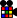Template:AlgAlg Algorithm

How to add algorithms:

1. Click the 'Edit' tab next to the case and you will have a editor for the wiki-code showing the text of the particular section you chose.
2. Copy-paste the last line that is an alg; {{Alg|R U R U}}, and change the alg to your one.
3. Click 'Show preview' and check everything is ok.
4. Click 'Save page' and you are done!

The template

This is an alg template that can be used to link algorithms to an animation and, optionally, a video. The template is very flexible, and its features and functions can be modified without changing the code of the algs on all the pages.

Examples:

Basic use:

{{Alg|R U R' U R U2' R'}}Alg R U R' U R U2' R'

If you want, you can specify that this is the alg. If not, the first parameter is assumed to be the alg.

{{Alg|alg=R U R' U R U2' R'}}Alg R U R' U R U2' R'

You can specify the stage of an alg, which is by default the second parameter:

{{Alg| R U' R' U2 R U' R' |stage=OLL}}

OR

{{Alg| R U' R' U2 R U' R' |OLL}}OLL RUR'URU2R'

Spacing in the alg does not matter:

{{Alg| R U R' U' R' F R2 U' R' U' R U R' F' | PLL}}PLL R U R' U' R' F R2 U' R' U' R U R' F'

You can link to a video (currently, only a single video):

{{Alg|R U R' U' R' F R2 U' R' U' R U R' F'|PLL|vidurl=http://www.youtube.com/watch?v=kD4SCn60jrc}}PLL R U R' U' R' F R2 U' R' U' R U R' F'